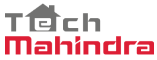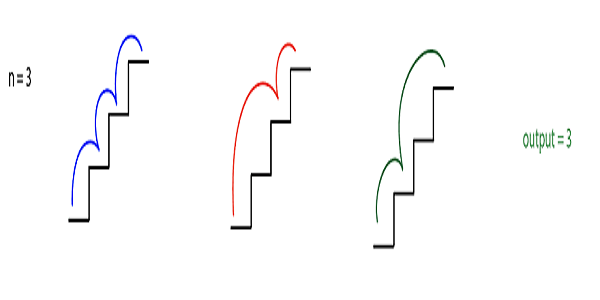New update is available. Click here to update.
Topics

# Count Ways To Reach The N-th Stairs

Moderate0/80
Average time to solve is 30m+44 more companies

## Problem statement

You have been given a number of stairs. Initially, you are at the 0th stair, and you need to reach the Nth stair. Each time you can either climb one step or two steps. You are supposed to return the number of distinct ways in which you can climb from the 0th step to Nth step.

Example :
``````N=3
````````````We can climb one step at a time i.e. {(0, 1) ,(1, 2),(2,3)} or we can climb the first two-step and then one step i.e. {(0,2),(1, 3)} or we can climb first one step and then two step i.e. {(0,1), (1,3)}.
``````
Detailed explanation ( Input/output format, Notes, Images )
Constraints :
``````1 <= 'T' <= 10
0 <= 'N' <= 10^5

Where 'T' is the number of test cases, and 'N' is the number of stairs.

It is guaranteed that sum of 'N' over all test cases is <= 10^5.
``````
Sample Input 1 :
``````2
2
3
``````
Sample Output 1 :
``````2
3
``````
Explanation Of Sample Input 1 :
``````In the first test case, there are only two ways to climb the stairs, i.e. {1,1} and {2}.

In the second test case, there are three ways to climb the stairs i.e. {1,1,1} , {1,2} and {2,1}.
``````
Sample Input 2 :
``````2
4
5
``````
Sample Output 2 :
``````5
8
``````
Explanation Of Sample Input 2 :
``````In the first test case, there are five ways to climb the stairs i.e. {1,1,1,1} , {1,1,2} , {2,1,1} , {1,2,1} , {2,2}.

In the second test case, there are eight ways to climb the stairs i.e. {1,1,1,1,1} , {1,1,1,2} , {1,1,2,1}, {1,2,1,1}, {2,1,1},{2,2,1},{2,1,2} and {2,2,1}.
``````Console